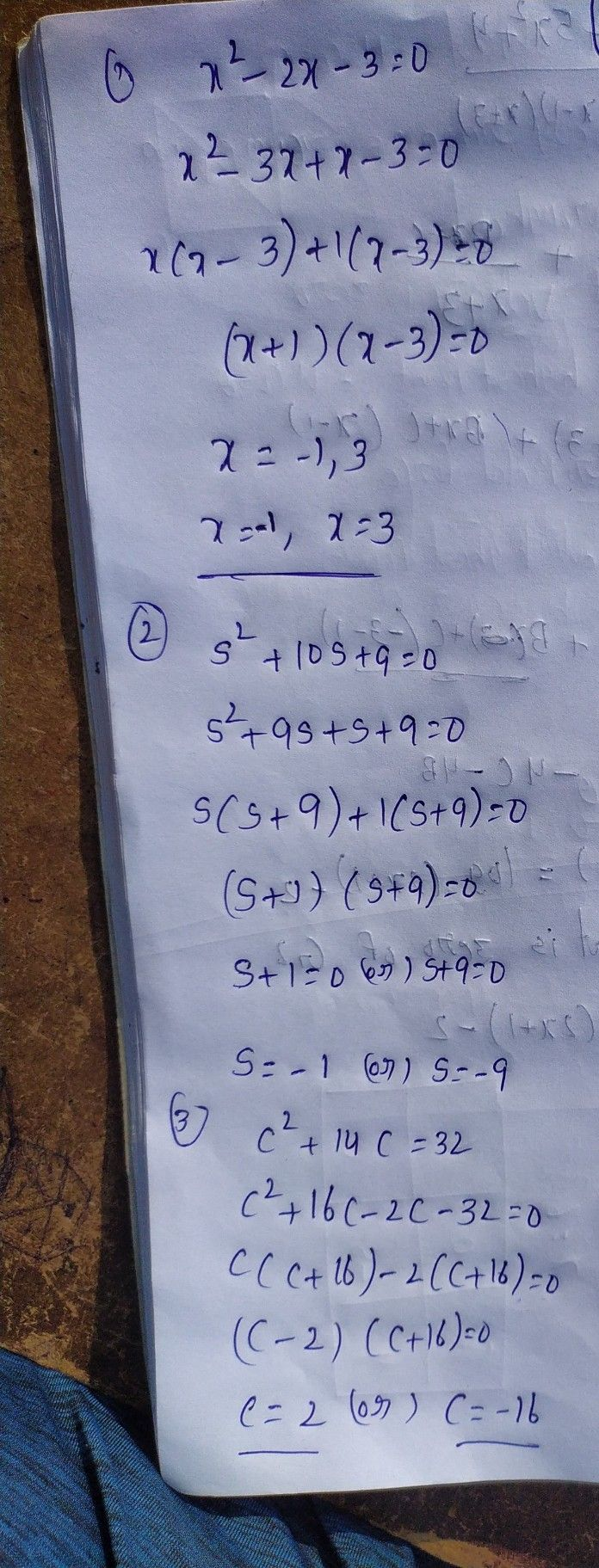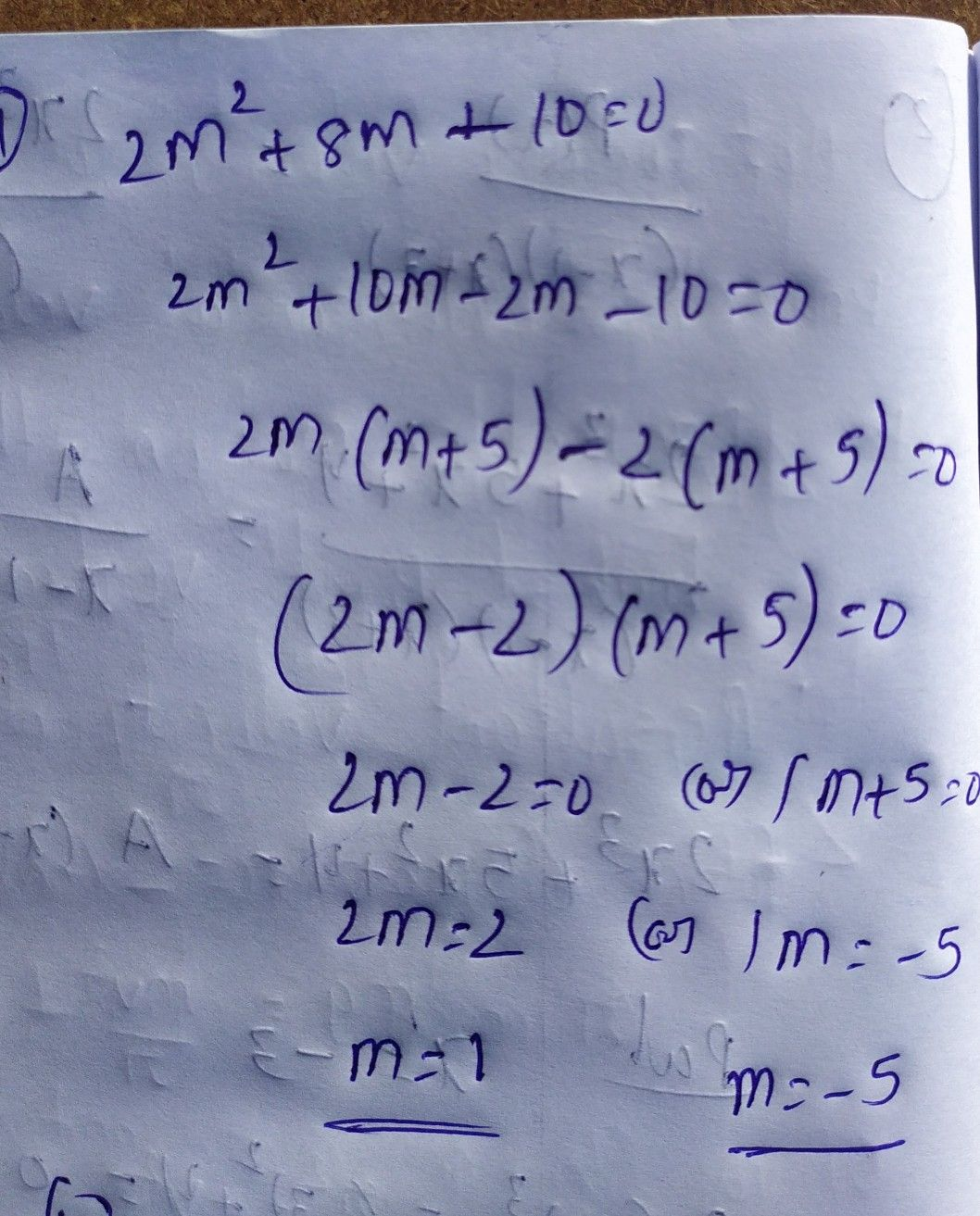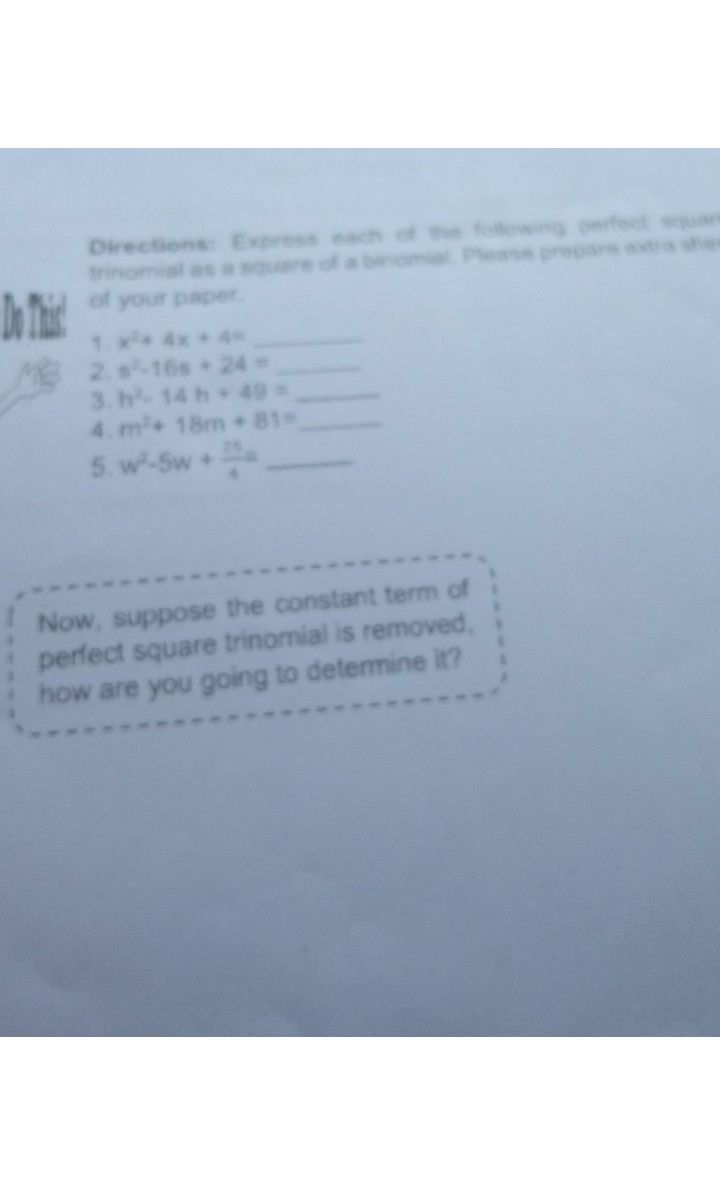Symbol
Problem$Directions:$ Find the solutions of each of the following quadratic equations by completing the square. Let's D his Answer the questions that follow. $1$ $x^{2}$ $-2x-3=0$ $2.$ $s^{2}+10s+9=0$ $3$ $c^{2}+14c=32$ $4$ $2m^{2}+8m-10=0$ $5$ $4b^{2}-32b=-28$ $Questions:$ a. How did you find the solutions of each equation? $1$ $2$ $3$ $4$ uin findi
1st-6th grade
Other
Search count: 111
SolutionQanda teacher - deekshithStudentQanda teacher - deekshith
please ask separately
please
understand